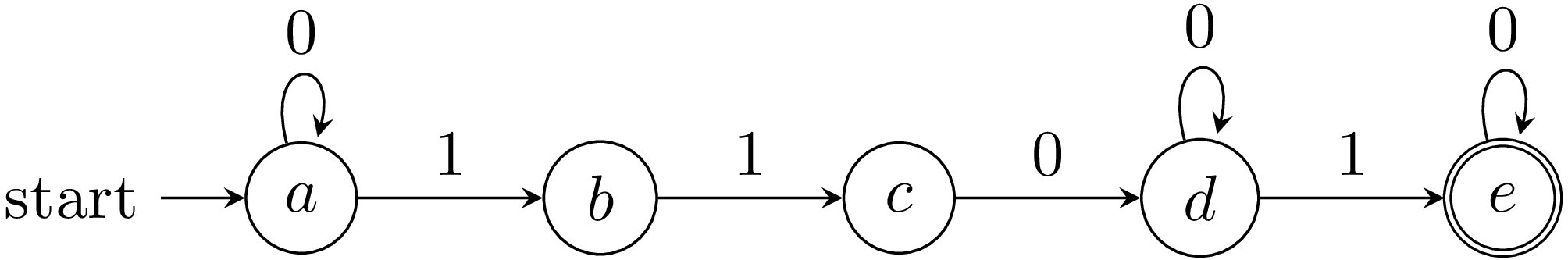PyCSP3

# Constraint Regular

A constraint Regular enforces the (sequence of) values assigned to the variables of a specified list to belong to a regular language specified by an automaton (i.e., forms a word that can be recognized by a deterministic, or non-deterministic, finite automaton). For such constraints, an automaton is then used to determine whether or not a given tuple is accepted. This can be an attractive approach when constraint relations can be naturally represented by regular expressions in a known regular language, or when a high level of compression (with respect to a classical table) is possible.

Let us recall that a Deterministic Finite Automaton (DFA) is a 5-tuple $(Q,\Sigma,\delta,q_0,F)$ where $Q$ is a finite set of states, $\Sigma$ is a finite set of symbols called the alphabet, $\delta:Q \times \Sigma \rightarrow Q$ is a transition function, $q_0 \in Q$ is the initial state, and $F \subseteq Q$ is the set of final states.

Given an input string (a finite sequence of symbols taken from the alphabet $\Sigma$), the automaton starts in the initial state $q_0$, and for each symbol in sequence of the string, applies the transition function to update the current state. If the last state reached is a final state then the input string is accepted by the automaton. The set of strings that the automaton $A$ accepts constitutes a language, denoted by $L(A)$, which is technically a regular language. When the automaton is non-deterministic, we can find two transitions $(q_i,a,q_j)$ and $(q_i,a,q_k)$ such that $q_j \neq q_k$.

Interestingly, in PyCSP$^3$, we can directly write constraints Regular in mathematical form, by using tuples, automatas and the operator ‘in’. The scope of a constraint is given by a tuple (or list) of variables on the left of the constraining expression and an automaton is given on the right of the constraining expression. Automatas are objects of the class Automaton that are built by specifying three named parameters, respectively denoting the initial state, the set of transitions and the set of final states.

These three required named parameters are:

• start is the name of the initial state (a string)
• transitions is a set (or list) of 3-tuples
• final is the set (or list) of the names of final states (strings)

Note that the set of states and the alphabet can be inferred from transitions.

To see how this constraint works, we need first to import the library PyCSP$^3$:

from pycsp3 import *


For our illustration, we introduce an array $x$ of 7 variables, each one with {0,1} as domain.

x = VarArray(size=7, dom={0,1})


We can display (the structure of) the array as well as the domain of the first variable.

print("Array of variable x: ", x)
print("Domain of any variable: ", x.dom)

Array of variable x:  [x, x, x, x, x, x, x]
Domain of any variable:  0 1


Our objective is to impose that the sequence of values assigned to the variables of $x$ belongs to the regular expression $0^1^20^+10^$. This corresponds to the following automaton:

The automaton $A$:We can represent this automaton $A$ as follows:

a, b, c, d, e = "a", "b", "c", "d", "e"  # names of states
t = [(a,0,a), (a,1,b), (b,1,c), (c,0,d), (d,0,d), (d,1,e), (e,0,e)]
A = Automaton(start=a, transitions=t, final=e)


We can print the textual representation of the automaton:

print(A)

Automaton(start=a, transitions={(a,0,a),(a,1,b),(b,1,c),(c,0,d),(d,0,d),(d,1,e),(e,0,e)}, final=[e])


We simply post a single constraint Regular on the variables of $x$ :

satisfy(
x in A
);


Interestingly, we can also display the internal representation of the posted constraint; although a little bit technical, we can see that the information is correctly recorded.

print(posted())

regular(list:[x, x, x, x, x, x, x], transitions:(a,0,a)(a,1,b)(b,1,c)(c,0,d)(d,0,d)(d,1,e)(e,0,e), start:a, final:[e])


By calling the function solve(), we can check that the problem (actually, the single constraint) is satisfiable (SAT). We can also print the values assigned to the variables in the found solution; we can call the function values() that collects the values assigned (in the last found solution) to a specified list of variables.

if solve() is SAT:
print("Solution: ", values(x))

Solution:  [0, 0, 0, 1, 1, 0, 1]


On can enumerate the solutions (supports) for this constraint as follows:

if solve(sols=ALL) is SAT:
for i in range(n_solutions()):
print(f"Solution {i+1}: {values(x, sol=i)}")

Solution 1: [0, 0, 0, 1, 1, 0, 1]
Solution 2: [0, 0, 1, 1, 0, 0, 1]
Solution 3: [0, 0, 1, 1, 0, 1, 0]
Solution 4: [0, 1, 1, 0, 0, 1, 0]
Solution 5: [0, 1, 1, 0, 0, 0, 1]
Solution 6: [0, 1, 1, 0, 1, 0, 0]
Solution 7: [1, 1, 0, 0, 1, 0, 0]
Solution 8: [1, 1, 0, 0, 0, 1, 0]
Solution 9: [1, 1, 0, 1, 0, 0, 0]
Solution 10: [1, 1, 0, 0, 0, 0, 1]